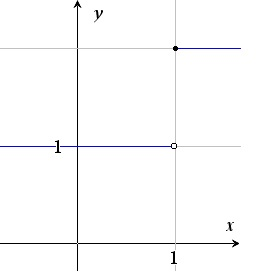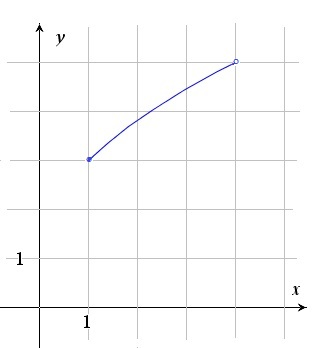# Various definitions

Up a level : IB Mathematics
Previous page : Functions, Quiz 1
Next page : Composite functionsThe : AB notation [SL/HL]

Sometimes we might see the notation : A : AB, B. This is read “f is a function from A to B” where A and B are some sets. We might for example have the function

f (x)=round x down to the nearest smaller integer

For this function we might write : RZ,  where R is the set of real numbers and Z is the set of integers.

Graphs of functions

A function can be regarded as a set of ordered pairs of numbers that can be seen as the coordinates of points. The function f (x)=x2 will, for example, contain the points (2, 4) and (3, 9). If we draw these points in a coordinate system we get a graph of the function.  The function y=f (x)=x2 will give us the graph:We usually use a Cartesian coordinate system, i.e. a system where the value of x is represented by the distance along one axis, usually the horizontal axis, and the value of y by the distance along a vertical axis, the axes being perpendicular (90o to each other) and  in this case drawn to the same scale.

If the function has steps, one can mark witch of the two possible values of the function is valid by marking it by a point, and by marking the other point by an unfilled circle as in the graph below.When the line just stops “mid air” then it indicates that it continues in some way.

Domain and Range

The domain of a function is the set of allowed input values, the “x”-values, and the range is the set of possible output values, the “y”-values.

My silly rule to remember this is to say “this is my domain (sweeping an arm out horizontally to indicate the x-values) and on it  I have a mountain range (sweeping an arm vertically to indicate the y-values). Yes, I know  it is silly – but it works.

Let us look a at the function described by the following graph:The domain is now the set of x-values such that 1≤x<4. This set can be written as [1,4) where the “[” indicates that the value next to it is included, and “)” indicates that the value is not included.

The range is now the set of y-values such that 3≤y<5, that can be written as [3,5).

For the f (x)=x2 the domain is all the real numbers, since we can square any real numbers. The range is y≥0, since the square of a real is always positive, but can be however big.

For the step function above we yet again have the domain being the real numbers (if we assume the graph goes on as straight lines in both directions). The range is then just the set {1, 2} since the only possible outputs are these two values.Up a level : IB Mathematics
Previous page : Functions, Quiz 1
Next page : Composite functionsLast modified: Jan 8, 2017 @ 15:08